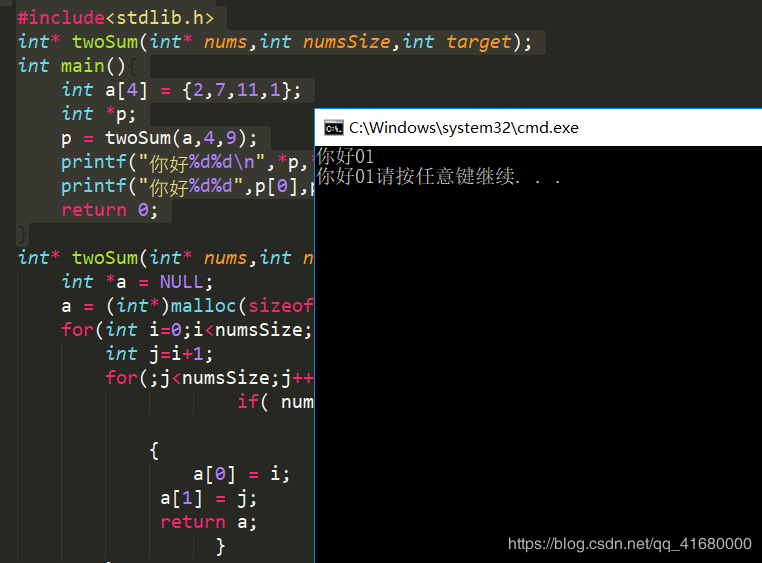# 给定一个整数数组 nums 和一个目标值 target，请你在该数组中找出和为目标值的那 两个 整数，并返回他们的数组下标。你可以假设每种输入只会对应一个答案。但是，你不能重复利用这个数组中同样的元素。

## 力扣通过代码

int* twoSum(int* nums, int numsSize, int target) {
int i=0;
int j;
int *a;
a = (int*)malloc(sizeof(int)*2);
for(;i<numsSize;i++){
j = i+1;
for(;j<numsSize;j++){
if( nums[i]+nums[j]==target)
{
a = i;
a = j;
return a;
}
}
}
return 0;
}


## 本机测试代码

#include<stdio.h>
#include<stdlib.h>
int* twoSum(int* nums,int numsSize,int target);
int main(){
int a = {2,7,11,1};
int *p;
p = twoSum(a,4,9);
printf("你好%d%d\n",*p,*(p+1));
printf("你好%d%d",p,p);
return 0;
}
int* twoSum(int* nums,int numsSize,int target) {//指针函数返回值为指针，函数指针返回值为函数
int *a = NULL;
a = (int*)malloc(sizeof(int)*2);
for(int i=0;i<numsSize;i++){
int j=i+1;
for(;j<numsSize;j++){
if( nums[i]+nums[j]==target)

{
a = i;
a = j;
return a;
}
}
}
return 0;
}


## 本机运行结果08-292万+07-248038
05-289299
10-21828
11-11126
01-20416
01-1123
03-06610
10-088732
02-254
02-2468
02-185
02-2515
10-234977
12-08
12-31
©️2020 CSDN 皮肤主题: 大白 设计师:CSDN官方博客